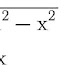In this article we are going to discuss about the velocity and acceleration of a particle performing simple harmonic motion. Velocity of a particle is defined as the time rate of change of displacement and similarly we define the term acceleration as the rate of change of velocity. Both the quantities are vectors that means there is always some directions associated with them.

As far as the case with linear simple harmonic motion is concerned, we know that it is the simplest type of oscillatory motion taking place in a straight line. Due to the rectilinear nature of SHM, all the vector terms associated with particle like velocity, acceleration, momentum cannot have more than two different directions (over the whole period of motion) and this fact eliminates the need to put an additional arrow sign along with the symbols of these quantities while representing them algebraically. Only plus (+) and minus (-) signs are sufficient to differentiate between the two possible directions.

As we have already discussed in our previous section named kinematics and dynamics of SHM that particle’s displacement is a sinusoidal function of time (in SHM).

x = Asin(ωt + Φ)               ...1

where A is the Amplitude of Oscillation, ω stands for the angular frequency and Φ for the phase constant or initial phase. 'x' represents displacement of particle from the mean position.

On respectively differentiating and double differentiating the displacement 'x' [from equation 1] with respect to time we can get the expression for velocity and acceleration.

v = Aωcos(ωt + Φ)          ...2

a = -Aω²sin(ωt + Φ)        ...3

The above two equations represent the sinusoidal variation of particle’s velocity and acceleration with time. From equation number 2, we can observe that the speed |v| will be maximum when cos(ωt + Φ) is maximum (i.e. equal to unity) and it will be minimum when modulus of cos(ωt + Φ) is minimum (i.e. equal to zero). Let's make some analysis based on equations 1, 2 & 3 by taking following two cases:

Case 1 : When Phase = 0

ωt + Φ = 0
∴  x = Asin0 = 0
v = Aωcos0 = Aω &
a = -Aω²sin0 = 0
Thus, when the particle crosses mean position, it's having maximum possible speed (equal to Aω) and minimum magnitude of acceleration (equal to zero).

Case 2 : When Phase = π/2

ωt + Φ = π/2

∴  x = Asin(π/2) = A

v = Aωcos(π/2) = 0  &

a = -Aω²sin(π/2) = -Aω²
Thus, when the particle reaches one of the extreme positions, its velocity is minimum (equal to zero) and acceleration is maximum in magnitude (equal to Aω²).

Velocity and Acceleration as functions of Position (x)

Observe the equation numbers 1, 2 & 3 written in the previous sub-section. Using them, we can derive the velocity-position and acceleration-position relationships.
On eliminating sin(ωt + Φ) from equations 1 & 3 we obtain the variation of acceleration with position as a = -ω²x. And again a similar operation on equations 1 & 2 yields velocity as function of position v² = ω (A² - x²).
In this way we have
From these two equations also we can again deduce:
0 < v <  Aω  and  0 < a <  Aω²
[ ∵ 0 < |x| < A]

Velocity and Acceleration in SHMReviewed by Raushan Raj on July 31, 2019 Rating: 5